# Modeling Target Radar Cross Section

This example shows how to model radar targets with increasing levels of fidelity. The example introduces the concept of radar cross sections (RCS) for simple point targets and extends it to more complicated cases of targets with multiple scattering centers. It also discusses how to model fluctuations of the RCS over time and briefly considers the case of polarized signals.

### Introduction

A radar system relies on target reflection or scattering to detect and identify targets. The more strongly a target reflects, the greater the returned echo at the radar receiver, resulting in a higher signal-to-noise ratio (SNR) and likelier detection. In radar systems, the amount of energy reflected from a target is determined by the radar cross section (RCS), defined aswhererepresents the RCS,is the distance between the radar and the target,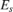is the field strength of the signal reflected from the target, andis the field strength of the signal incident on the target. In general, targets scatter energy in all directions and the RCS is a function of the incident angle, the scattering angle, and the signal frequency. RCS depends on the shape of the target and the materials from which it is constructed. Common units used for RCS include square meters or dBsm.

This example focuses on narrowband monostatic radar systems, when the transmitter and receiver are co-located. The incident and scattered angles are equal and the RCS is a function only of the incident angle. This is the backscattered case. For a narrowband radar, the signal bandwidth is small compared to the operating frequency and is therefore considered to be constant.

### RCS of a Simple Point Target

The simplest target model is an isotropic scatterer. An example of an isotropic scatterer is a metallic sphere of uniform density. In this case, the reflected energy is independent of the incident angle. An isotropic scatterer can often serve as a first order approximation of a more complex point target that is distant from the radar. For example, a pedestrian can be approximated by an isotropic scatterer with a 1 square meter RCS.

c = 3e8; fc = 3e8; pedestrian = phased.RadarTarget('MeanRCS',1,'PropagationSpeed',c,... 'OperatingFrequency',fc) 
pedestrian = phased.RadarTarget with properties: EnablePolarization: false MeanRCSSource: 'Property' MeanRCS: 1 Model: 'Nonfluctuating' PropagationSpeed: 300000000 OperatingFrequency: 300000000 

where c is the propagation speed and fc is the operating frequency of the radar system. The scattered signal from a unit input signal can then be computed as

x = 1; ped_echo = pedestrian(x) 
ped_echo = 3.5449 

where x is the incident signal. The relation between the incident and the reflected signal can be expressed as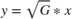where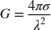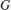represents the dimensionless gain that results from the target reflection.is the wavelength corresponding to the system's operating frequency.

### RCS of Complex Targets

For targets with more complex shapes, reflections can non longer be considered the same across all directions. The RCS varies with the incident angles (also known as aspect angles). Aspect-dependent RCS patterns can be measured or modeled just as you would antenna radiation patterns. The result of such measurements or models is a table of RCS values as a function of azimuth and elevation angles in the target's local coordinate system.

The example below first computes the RCS pattern of a cylindrical target, with a radius of 1 meter and a height of 10 meters, as a function of azimuth and elevation angles.[cylrcs,az,el] = rcscylinder(1,1,10,c,fc); 

Because the cylinder is symmetric around the z axis, there is no azimuth-angle dependency. RCS values vary only with elevation angle.

helperTargetRCSPatternPlot(az,el,cylrcs); title('RCS Pattern of Cylinder');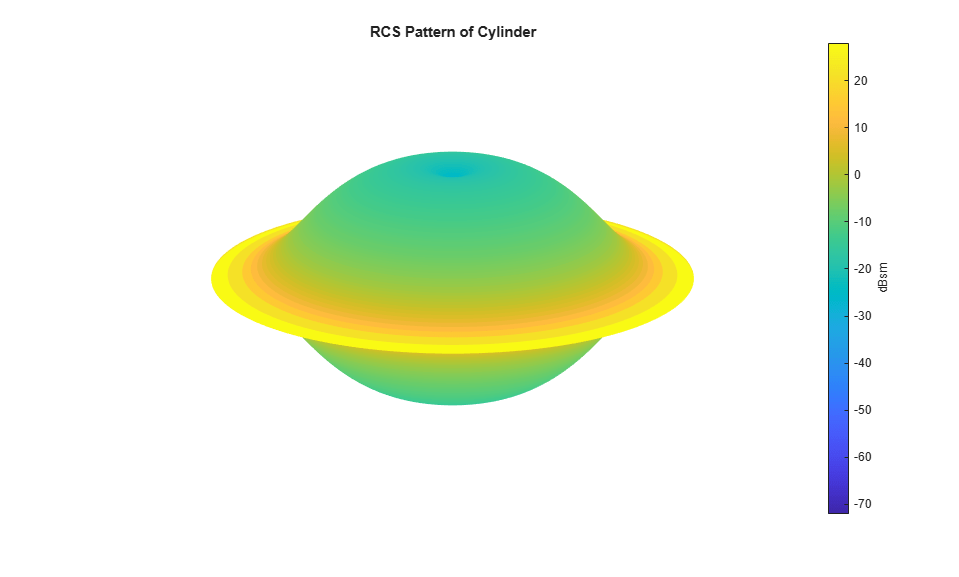The pattern in the elevation cut looks like

plot(el,pow2db(cylrcs)); grid; axis tight; ylim([-30 30]); xlabel('Elevation Angles (degrees)'); ylabel('RCS (dBsm)'); title('RCS Pattern for Cylinder');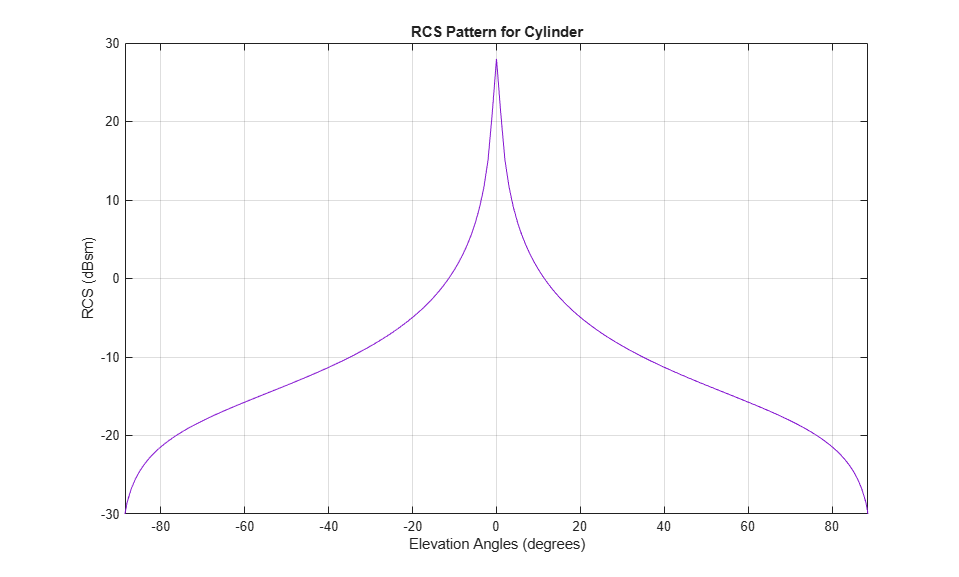The aspect-dependent RCS patterns can then imported into a phased.BackscatterRadarTarget object.

cylindricalTarget = phased.BackscatterRadarTarget('PropagationSpeed',c,... 'OperatingFrequency',fc,'AzimuthAngles',az,'ElevationAngles',el,... 'RCSPattern',cylrcs) 
cylindricalTarget = phased.BackscatterRadarTarget with properties: EnablePolarization: false AzimuthAngles: [-180 -179 -178 -177 -176 -175 -174 -173 -172 ... ] ElevationAngles: [-90 -89 -88 -87 -86 -85 -84 -83 -82 -81 -80 -79 ... ] RCSPattern: [181x361 double] Model: 'Nonfluctuating' PropagationSpeed: 300000000 OperatingFrequency: 300000000 

Finally, generate the target reflection. Assume three equal signals are reflected from the target at three different aspect angles. The first two angles have the same elevation angle but with different azimuth angles. The last has a different elevation angle from the first two.

x = [1 1 1]; % 3 unit signals ang = [0 30 30;0 0 30]; % 3 directions cyl_echo = cylindricalTarget(x,ang) 
cyl_echo = 88.8577 88.8577 1.3161 

One can verify that there is no azimuth angle dependence because the first two outputs are the same.

The number of target shapes for which analytically-derived RCS patterns exist are few. For more complicated shapes and materials, computational electromagnetics approaches, such as method of moments (MoM), or finite element analysis (FEM), can be used to accurately predict the RCS pattern. A more detailed discussion of these techniques is available in . You can use the output of these computations as input to the phased.BackscatterRadarTarget System object™ as was done in the cylinder example before.

### RCS of Extended Targets with Multiple Scatterers

Although computational electromagnetic approaches can provide accurate RCS predictions, they often require a significant amount of computation and are not suitable for real-time simulations. An alternative approach for describing a complex targets is to model it as a collection of simple scatterers. The RCS pattern of the complex target can then be derived from the RCS patterns of the simple scatterer aswhereis the RCS of the target,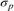is the RCS of theth scatterer, andis the relative phase of theth scatterer. A multi-scatterer target behaves much like an antenna array.

The next section shows how to model a target consisting of four scatterers. The scatterers are located at the four vertices of a square. Each scatterer is a cylindrical point target as derived in the previous section. Without loss of generality, the square is placed in the xy -plane. The side length of the square is 0.5 meter.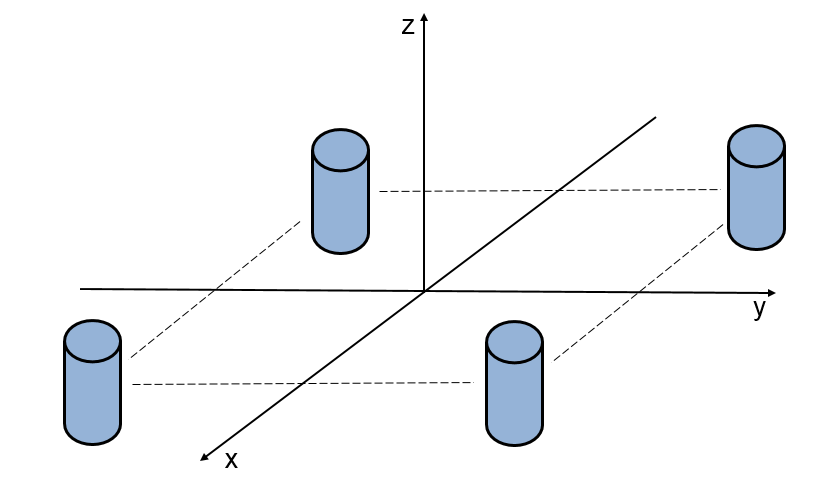First, define the positions of the scatterers.

scatpos = [-0.5 -0.5 0.5 0.5;0.5 -0.5 0.5 -0.5;0 0 0 0]; 

If the target is in the far field of the transmitter, the incident angle for each component scatterer is the same. Then, the total RCS pattern can be computed as

naz = numel(az); nel = numel(el); extrcs = zeros(nel,naz); for m = 1:nel sv = steervec(scatpos,[az;el(m)*ones(1,naz)]); % sv is squared due to round trip in a monostatic scenario extrcs(m,:) = abs(sqrt(cylrcs(m,:)).*sum(sv.^2)).^2; end 

The total RCS pattern then looks like

helperTargetRCSPatternPlot(az,el,extrcs); title('RCS Pattern of Extended Target with 4 Scatterers');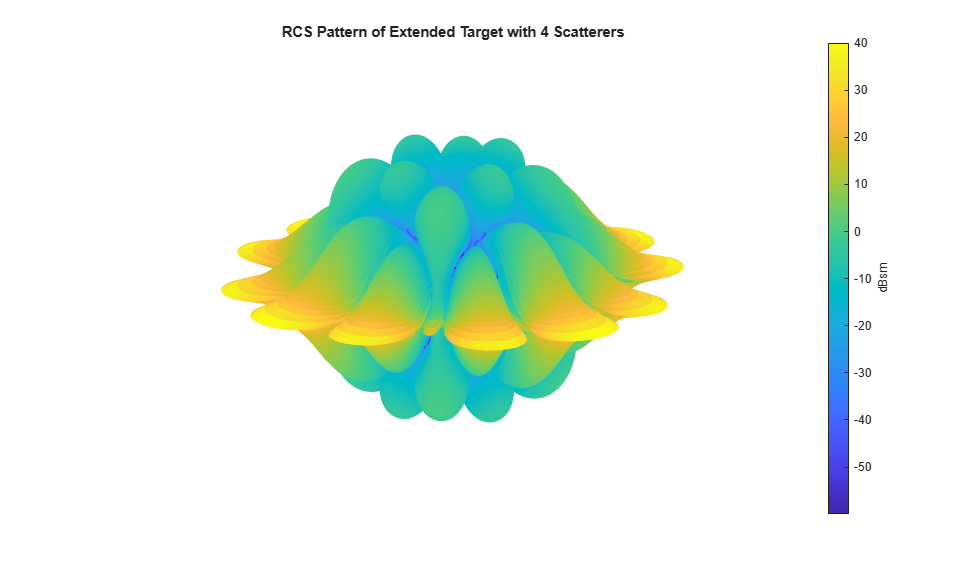This pattern can then be used in a phased.BackscatterRadarTarget object to compute the reflected signal. The results verify that the reflected signal depends on both azimuth and elevation angles.

extendedTarget = phased.BackscatterRadarTarget('PropagationSpeed',c,... 'OperatingFrequency',fc,'AzimuthAngles',az,'ElevationAngles',el,... 'RCSPattern',extrcs); ext_echo = extendedTarget(x,ang) 
ext_echo = 355.4306 236.7633 0.0000 

### Wideband RCS of Extended Targets with Multiple Scatterers

Wideband radar systems are typically defined as having a bandwidth greater than 5% of their center frequency. In addition to improved range resolution, wideband systems also offer improved target detection. One way in which wideband systems improve detection performance is by filling in fades in a target's RCS pattern. This can be demonstrated by revisiting the extended target comprised of 4 cylindrical scatterers used in the preceding section. The modeled narrowband RCS swept across various target aspects is shown as

sweepaz = -90:90; % Azimuthal sweep across target sweepel = 0; [elg,azg] = meshgrid(sweepel,sweepaz); sweepang = [azg(:)';elg(:)']; x = ones(1,size(sweepang,2)); % unit signals release(extendedTarget); extNarrowbandSweep = extendedTarget(x,sweepang); clf; plot(sweepaz,pow2db(extNarrowbandSweep)); grid on; axis tight; xlabel('Azimuth Angles (degrees)'); ylabel('RCS (dBsm)'); title(['RCS Pattern at 0^o Elevation ',... 'for Extended Target with 4 Scatterers']);Returns from the multiple cylinders in the extended target model coherently combine, creating deep fades between 40 and 50 degrees. These fades can cause the target to not be detected by the radar sensor.

Next, the RCS pattern for a wideband system operating at the same center frequency will be examined. The bandwidth for this system will be set to 10% of the center frequency

bw = 0.10*fc; % Bandwidth is greater-than 5% of center frequency fs = 2*bw; 

A wideband RCS model is created as was previously done for the narrowband extended target. Often, RCS models are generated offline using either simulation tools or range measurements and are then provided to radar engineers for use in their system models. Here, it is assumed that the provided RCS model has been sampled at 1MHz intervals on either side of the radar's center frequency.

modelFreq = (-80e6:1e6:80e6)+fc; [modelCylRCS,modelAz,modelEl] = helperCylinderRCSPattern(c,modelFreq); 

The contributions from the various scattering centers are modeled as before. It is important to note that this approximation assumes that all of the target's scattering centers fall within the same range resolution bin, which is true for this example.

nf = numel(modelFreq); naz = numel(modelAz); nel = numel(modelEl); modelExtRCS = zeros(nel,naz,nf); for k = 1:nf for m = 1:nel pos = scatpos*modelFreq(k)/fc; sv = steervec(pos,[modelAz;modelEl(m)*ones(1,naz)]); % sv is squared due to round trip in a monostatic scenario modelExtRCS(m,:,k) = abs(sqrt(modelCylRCS(m,:,k)).*sum(sv.^2)).^2; end end 

The wideband RCS target model is now generated, using the RCS patterns that were just computed.

widebandExtendedTarget = phased.WidebandBackscatterRadarTarget(... 'PropagationSpeed',c,'OperatingFrequency',fc,'SampleRate',fs,... 'AzimuthAngles',modelAz,'ElevationAngles',modelEl,... 'FrequencyVector',modelFreq,'RCSPattern',modelExtRCS); 

The modeled wideband RCS can now be compared to the narrowband system

extWidebandSweep = widebandExtendedTarget(x,sweepang); hold on; plot(sweepaz,pow2db(extWidebandSweep)); hold off; legend('Narrowband','Wideband');The target's RCS pattern now has much shallower nulls between 40 and 50 degrees azimuth. The deep nulls in the narrowband pattern occur when signals combine destructively at a specific frequency and azimuth combination. The wideband waveform fills in these fades because, while a few frequencies may experience nulls for a given aspect, the majority of the bandwidth does not lie within the null at that azimuth angle.

### RCS of Fluctuating Targets

The discussion so far assumes that the target RCS value is constant over time. This is the nonfluctuating target case. In reality, because both the radar system and the target are moving, the RCS value changes over time. This case is a fluctuating target. To simulate fluctuating targets, Peter Swerling developed four statistical models, referred to as Swerling 1 through Swerling 4, that are widely adopted in practice. The Swerling models divide fluctuating targets into two probability distributions and two time varying behaviors as shown in the following table:

 Slow Fluctuating Fast Fluctuating ----------------------------------------------------------------- Exponential Swerling 1 Swerling 2 4th Degree Chi-square Swerling 3 Swerling 4

The RCS of a slow-fluctuating target remains constant during a dwell but varies from scan to scan. In contrast, the RCS for a fast fluctuating target changes with each pulse within a dwell.

The Swerling 1 and 2 models obey an exponential density function (pdf) given by,

These models are useful in simulating a target consisting of a collection of equal strength scatterers.

The Swerling 3 and 4 models obey a 4th degree Chi-square pdf, given by,

These models apply when the target contains a dominant scattering component. In both pdf definitions,represents the mean RCS value, which is the RCS value of the same target under the nonfluctuating assumption.

The next section shows how to apply a Swerling 1 statistical model when generating the radar echo from the previously described cylindrical target.

cylindricalTargetSwerling1 = ... phased.BackscatterRadarTarget('PropagationSpeed',c,... 'OperatingFrequency',fc,'AzimuthAngles',az,'ElevationAngles',el,... 'RCSPattern',cylrcs,'Model','Swerling1') 
cylindricalTargetSwerling1 = phased.BackscatterRadarTarget with properties: EnablePolarization: false AzimuthAngles: [-180 -179 -178 -177 -176 -175 -174 -173 -172 ... ] ElevationAngles: [-90 -89 -88 -87 -86 -85 -84 -83 -82 -81 -80 -79 ... ] RCSPattern: [181x361 double] Model: 'Swerling1' PropagationSpeed: 300000000 OperatingFrequency: 300000000 SeedSource: 'Auto' 

In the Swerling 1 case, the reflection is no longer constant. The RCS value varies from scan to scan. Assuming that the target is illuminated by the signal only once per dwell, the following code simulates the reflected signal power for 10,000 scans for a unit incident signal.

N = 10000; tgt_echo = zeros(1,N); x = 1; for m = 1:N tgt_echo(m) = cylindricalTargetSwerling1(x,[0;0],true); end p_echo = tgt_echo.^2; % Reflected power 

Plot the histogram of returns from all scans and verify that the distribution of the returns match the theoretical prediction. The theoretical prediction uses the nonfluctuating RCS derived before. For the cylindrical target, the reflected signal power at normal incidence for unit power input signal is

p_n = cyl_echo(1)^2; helperTargetRCSReturnHistogramPlot(p_echo,p_n)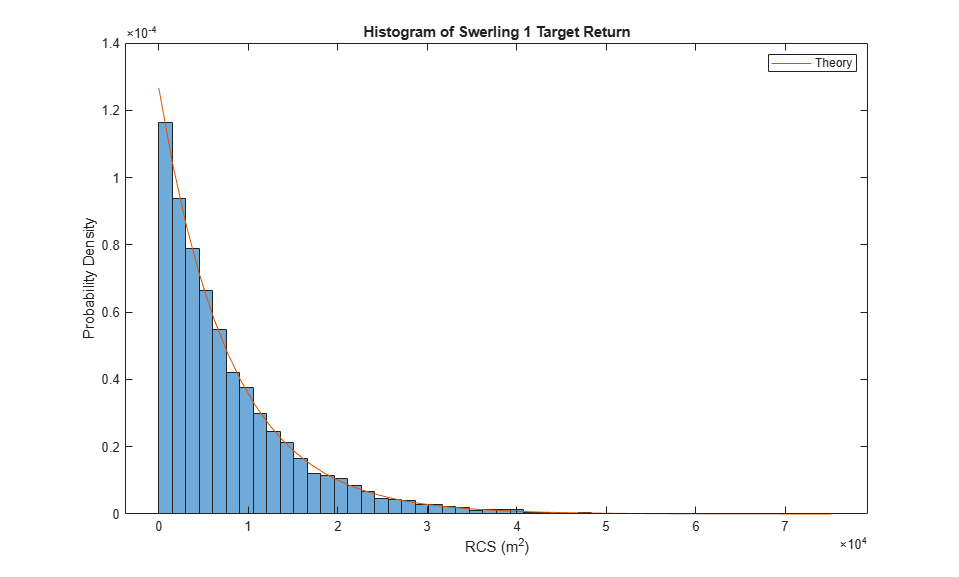### RCS of Polarized Targets

The target RCS is also a function of polarization. To describe the polarization signature of a target, a single RCS value is no longer sufficient. Instead, for each frequency and incident angle, a scattering matrix is used to describe the interaction of the target with the incoming signal's polarization components. This example will not go into further details because this topic is covered in the Modeling and Analyzing Polarization example.

### Conclusion

This example gave a brief introduction to radar target modeling for a radar system simulation. It showed how to model point targets, targets with measured patterns, and extended targets. It also described how to take statistical fluctuations into account when generating target echoes.

### Reference

 Merrill Skolnik, Radar Handbook, 2nd Ed. Chapter 11, McGraw-Hill, 1990

 Bassem Mahafza, Radar Systems Analysis and Design Using MATLAB, 2nd Ed. Chapman & Hall/CRC, 2005